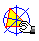ANTIPEDAL LINE

Let P be a point on the circumcircle of ABC and P* its isogonal point. The line PP* is the antipedal line of P. As P* is an infinite point, the antipedal line can be constructed as the parallel at P to the symmetrical line of AP with respect to the bisector of the angle A.Antipedal_line.mac